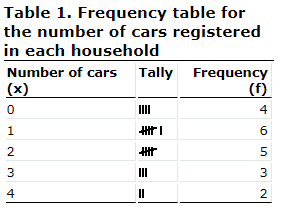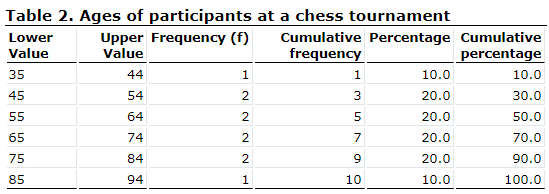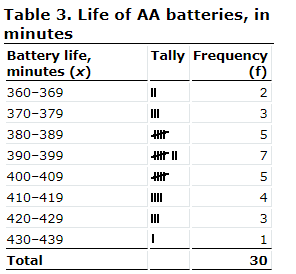Courses

# Construction of a Frequency Distribution, Business Mathematics & Statistics B Com Notes | EduRev

## B Com : Construction of a Frequency Distribution, Business Mathematics & Statistics B Com Notes | EduRev

The document Construction of a Frequency Distribution, Business Mathematics & Statistics B Com Notes | EduRev is a part of the B Com Course Business Mathematics and Statistics.
All you need of B Com at this link: B Com

## Frequency distribution tables

The frequency (f) of a particular observation is the number of times the observation occurs in the data. The distribution of a variable is the pattern of frequencies of the observation. Frequency distributions are portrayed as frequency tables, histograms, or polygons.

Frequency distributions can show either the actual number of observations falling in each range or the percentage of observations. In the latter instance, the distribution is called a relative frequency distribution.

Frequency distribution tables can be used for both categorical and numeric variables. Continuous variables should only be used with class intervals, which will be explained shortly

Example 1 – Constructing a frequency distribution table

A survey was taken on Maple Avenue. In each of 20 homes, people were asked how many cars were registered to their households. The results were recorded as follows:

1, 2, 1, 0, 3, 4, 0, 1, 1, 1, 2, 2, 3, 2, 3, 2, 1, 4, 0, 0

Use the following steps to present this data in a frequency distribution table.

1. Divide the results (x) into intervals, and then count the number of results in each interval. In this case, the intervals would be the number of households with no car (0), one car (1), two cars (2) and so forth.
2. Make a table with separate columns for the interval numbers (the number of cars per household), the tallied results, and the frequency of results in each interval. Label these columns Number of carsTally and Frequency.
3. Read the list of data from left to right and place a tally mark in the appropriate row. For example, the first result is a 1, so place a tally mark in the row beside where 1 appears in the interval column (Number of cars). The next result is a 2, so place a tally mark in the row beside the 2, and so on. When you reach your fifth tally mark, draw a tally line through the preceding four marks to make your final frequency calculations easier to read.
4. Add up the number of tally marks in each row and record them in the final column entitled Frequency.

Your frequency distribution table for this exercise should look like this:By looking at this frequency distribution table quickly, we can see that out of 20 households surveyed, 4 households had no cars, 6 households had 1 car, etc.

Example 2 – Constructing a cumulative frequency distribution table

cumulative frequency distribution table is a more detailed table. It looks almost the same as a frequency distribution table but it has added columns that give the cumulative frequency and the cumulative percentage of the results, as well.

At a recent chess tournament, all 10 of the participants had to fill out a form that gave their names, address and age. The ages of the participants were recorded as follows:

36, 48, 54, 92, 57, 63, 66, 76, 66, 80

Use the following steps to present these data in a cumulative frequency distribution table.

1. Divide the results into intervals, and then count the number of results in each interval. In this case, intervals of 10 are appropriate. Since 36 is the lowest age and 92 is the highest age, start the intervals at 35 to 44 and end the intervals with 85 to 94.
2. Create a table similar to the frequency distribution table but with three extra columns.
• In the first column or the Lower value column, list the lower value of the result intervals. For example, in the first row, you would put the number 35.
• The next column is the Upper value column. Place the upper value of the result intervals. For example, you would put the number 44 in the first row.
• The third column is the Frequency column. Record the number of times a result appears between the lower and upper values. In the first row, place the number 1.
• The fourth column is the Cumulative frequency column. Here we add the cumulative frequency of the previous row to the frequency of the current row. Since this is the first row, the cumulative frequency is the same as the frequency. However, in the second row, the frequency for the 35–44 interval (i.e., 1) is added to the frequency for the 45–54 interval (i.e., 2). Thus, the cumulative frequency is 3, meaning we have 3 participants in the 34 to 54 age group.

1 + 2 = 3

• The next column is the Percentage column. In this column, list the percentage of the frequency. To do this, divide the frequency by the total number of results and multiply by 100. In this case, the frequency of the first row is 1 and the total number of results is 10. The percentage would then be 10.0.

10.0. (1 ÷ 10) X 100 = 10.0

• The final column is Cumulative percentage. In this column, divide the cumulative frequency by the total number of results and then to make a percentage, multiply by 100. Note that the last number in this column should always equal 100.0. In this example, the cumulative frequency is 1 and the total number of results is 10, therefore the cumulative percentage of the first row is 10.0.

10.0. (1 ÷ 10) X 100 = 10.0

3. The cumulative frequency distribution table should look like this:

4.For more information on how to make cumulative frequency tables, see the section on Cumulative frequency and Cumulative percentage.

Class intervals

If a variable takes a large number of values, then it is easier to present and handle the data by grouping the values into class intervals. Continuous variables are more likely to be presented in class intervals, while discrete variables can be grouped into class intervals or not.

To illustrate, suppose we set out age ranges for a study of young people, while allowing for the possibility that some older people may also fall into the scope of our study.

The frequency of a class interval is the number of observations that occur in a particular predefined interval. So, for example, if 20 people aged 5 to 9 appear in our study's data, the frequency for the 5–9 interval is 20.

The endpoints of a class interval are the lowest and highest values that a variable can take. So, the intervals in our study are 0 to 4 years, 5 to 9 years, 10 to 14 years, 15 to 19 years, 20 to 24 years, and 25 years and over. The endpoints of the first interval are 0 and 4 if the variable is discrete, and 0 and 4.999 if the variable is continuous. The endpoints of the other class intervals would be determined in the same way.

Class interval width is the difference between the lower endpoint of an interval and the lower endpoint of the next interval. Thus, if our study's continuous intervals are 0 to 4, 5 to 9, etc., the width of the first five intervals is 5, and the last interval is open, since no higher endpoint is assigned to it. The intervals could also be written as 0 to less than 5, 5 to less than 10, 10 to less than 15, 15 to less than 20, 20 to less than 25, and 25 and over.

### Rules for data sets that contain a large number of observations

In summary, follow these basic rules when constructing a frequency distribution table for a data set that contains a large number of observations:

• find the lowest and highest values of the variables
• decide on the width of the class intervals
• include all possible values of the variable.

In deciding on the width of the class intervals, you will have to find a compromise between having intervals short enough so that not all of the observations fall in the same interval, but long enough so that you do not end up with only one observation per interval.

It is also important to make sure that the class intervals are mutually exclusive.

Example 3 – Constructing a frequency distribution table for large numbers of observations

Thirty AA batteries were tested to determine how long they would last. The results, to the nearest minute, were recorded as follows:

423, 369, 387, 411, 393, 394, 371, 377, 389, 409, 392, 408, 431, 401, 363, 391, 405, 382, 400, 381, 399, 415, 428, 422, 396, 372, 410, 419, 386, 390

Use the steps in Example 1 and the above rules to help you construct a frequency distribution table.

The lowest value is 363 and the highest is 431.

Using the given data and a class interval of 10, the interval for the first class is 360 to 369 and includes 363 (the lowest value). Remember, there should always be enough class intervals so that the highest value is included.

The completed frequency distribution table should look like this:Relative frequency and percentage frequency

An analyst studying these data might want to know not only how long batteries last, but also what proportion of the batteries falls into each class interval of battery life.

This relative frequency of a particular observation or class interval is found by dividing the frequency (f) by the number of observations (n): that is, (f ÷ n). Thus:

Relative frequency = frequency ÷ number of observations

The percentage frequency is found by multiplying each relative frequency value by 100. Thus:

Percentage frequency = relative frequency X 100 = f ÷ n X 100

## Example 4 – Constructing relative frequency and percentage frequency tables

Use the data from Example 3 to make a table giving the relative frequency and percentage frequency of each interval of battery life.

Here is what that table looks like:An analyst of these data could now say that:

• 7% of AA batteries have a life of from 360 minutes up to but less than 370 minutes, and that
• the probability of any randomly selected AA battery having a life in this range is approximately 0.07.

Keep in mind that these analytical statements have assumed that a representative sample was drawn. In the real world, an analyst would also refer to an estimate of variability (see section titled Measures of spread) to complete the analysis. For our purpose, however, it is enough to know that frequency distribution tables can provide important information about the population from which a sample was drawn.

Offer running on EduRev: Apply code STAYHOME200 to get INR 200 off on our premium plan EduRev Infinity!

122 videos|142 docs

,

,

,

,

,

,

,

,

,

,

,

,

,

,

,

,

,

,

,

,

,

,

,

,

;